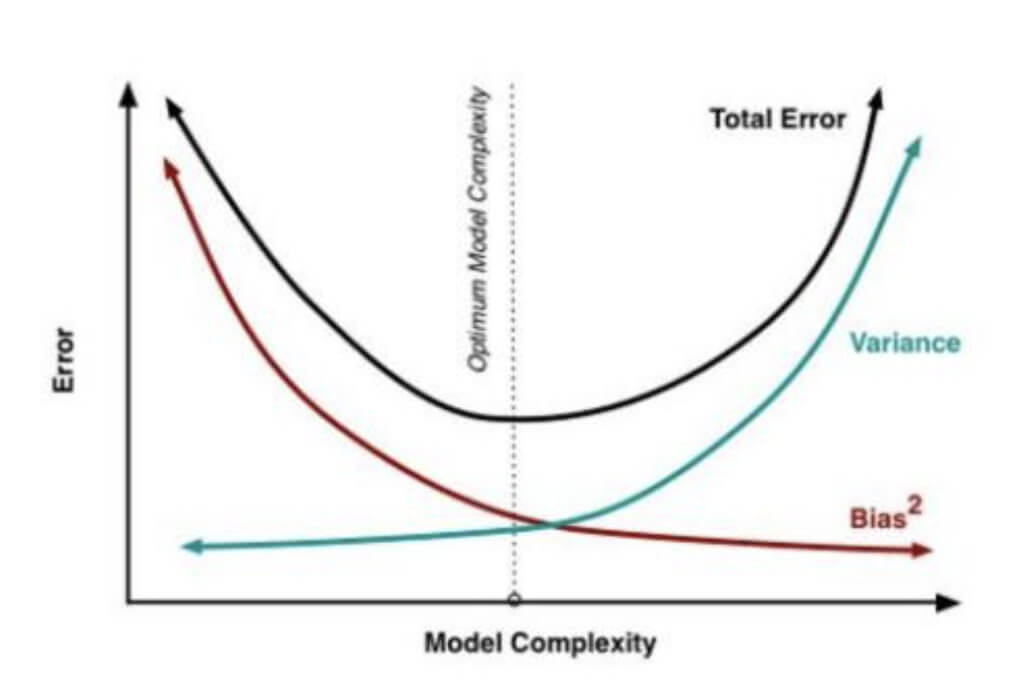# 【机器学习基础】第五课：偏差与方差

## 偏差-方差分解，偏差，方差，噪声，偏差-方差窘境，代价的类型

Posted by x-jeff on April 17, 2019

【机器学习基础】系列博客为参考周志华老师的《机器学习》一书，自己所做的读书笔记。

# 1.偏差-方差分解

$x$ 测试样本
$D$ 训练集
$y_D$ $x$在数据集中的标记
$y$ $x$的真实标记
$f$ 训练集$D$学得的模型
$f(x;D)$ 由训练集$D$学得的模型$f$对$x$的预测输出
$\overline{f}(x)$ 模型$f$对$x$的期望预测输出

⚠️有可能出现噪声使得$y_D\neq y$。

👉以回归任务为例：

$\overline{f}(x)=\mathbb{E}_D[f(x;D)]$

$var(x)=\mathbb{E}_D[(f(x;D)-\overline{f}(x))^2]$

$\varepsilon^2=\mathbb{E}_D[(y_D-y)^2]$

$bias^2(x)=(\overline{f}(x)-y)^2$

\begin{align} E(f;D) & = \mathbb{E}_D[(f(x;D)-y_D)^2] \\ & = \mathbb{E}_D[(f(x;D)-\overline{f}(x)+\overline{f}(x)-y_D)^2] \\ & = \mathbb{E}_D[(f(x;D)-\overline{f}(x))^2] + \mathbb{E}_D[(\overline{f}(x)-y_D)^2]+\mathbb{E}_D[2(f(x;D)-\overline{f}(x))(\overline{f}(x)-y_D)] \\ & = \mathbb{E}_D[(f(x;D)-\overline{f}(x))^2]+\mathbb{E}_D[(\overline{f}(x)-y_D)^2] \\ & = \mathbb{E}_D[(f(x;D)-\overline{f}(x))^2] +\mathbb{E}_D[(\overline{f}(x)-y+y-y_D)^2] \\ & = \mathbb{E}_D[(f(x;D)-\overline{f}(x))^2]+\mathbb{E}_D[(\overline{f}(x)-y)^2]+\mathbb{E}_D[(y-y_D)^2]+2\mathbb{E}_D[(\overline{f}(x)-y)(y-y_D)] \\ & = \mathbb{E}_D[(f(x;D)-\overline{f}(x))^2] +(\overline{f}(x)-y)^2+\mathbb{E}_D[(y_D-y)^2] \\ & = var(x)+bias^2(x)+\varepsilon^2 \end{align}

$\mathbb{E}_D [ 2(f(x;D)-\bar{f}(x)) (\bar{f}(x)-y_D)]=\mathbb{E}_D[2(f(x;D)-\bar{f}(x)) \cdot \bar{f}(x)] - \mathbb{E}_D[2(f(x;D)-\bar{f}(x))\cdot y_D] \tag{1.1}$

👉化简式(1.1)的第一项：

$\mathbb{E}_D[2(f(x;D)-\bar{f}(x)) \cdot \bar{f}(x)] = \mathbb{E}_D[2f(x;D)\cdot \bar{f}(x)-2\bar{f}(x)\cdot \bar{f}(x)]$

$\mathbb{E}_D[2(f(x;D)-\bar{f}(x)) \cdot \bar{f}(x)]=2\bar{f}(x) \cdot \mathbb{E}_D[f(x;D)]-2\bar{f}(x)\cdot \bar{f}(x)$

👉化简式(1.1)的第二项：

$\mathbb{E}_D[2(f(x;D)-\bar{f}(x))\cdot y_D] =2\mathbb{E}_D [f(x;D)\cdot y_D] - 2\bar{f}(x) \cdot \mathbb{E}_D[y_D]$

\begin{align} \mathbb{E}_D[2(f(x;D)-\bar{f}(x))\cdot y_D] &=2\mathbb{E}_D [f(x;D)\cdot y_D] - 2\bar{f}(x) \cdot \mathbb{E}_D[y_D] \\&= 2\mathbb{E}_D[f(x;D)]\cdot \mathbb{E}_D[y_D] - 2\bar{f}(x) \cdot \mathbb{E}_D[y_D] \\&= 2\bar{f}(x)\cdot \mathbb{E}_D[y_D] - 2\bar{f}(x) \cdot \mathbb{E}_D[y_D] \\&= 0\end{align}

❗️也就是说，泛化误差可分解为偏差、方差与噪声之和。

⚠️偏差-方差分解，这样优美的形式仅在基于均方误差的回归任务中得以推导出。对于分类任务，由于0/1损失函数的跳变性，理论上推导出偏差-方差分解很困难。

👉偏差：

👉方差：

👉噪声：

# 3.偏差-方差窘境👉很多学习算法都可控制训练程度，例如决策树可控制层数，神经网络可控制训练轮数，集成学习方法可控制基学习器个数。

# 5.参考资料

1. 泛化误差与偏差、方差的关系示意图来源：知乎：为什么xgboost/gbdt在调参时为什么树的深度很少就能达到很高的精度？—用户“于菲”的回答
2. 南瓜书PumpkinBook